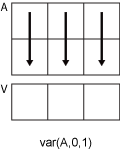# var

## Syntax

``V = var(A)``
``V = var(A,w)``
``V = var(A,w,'all')``
``V = var(A,w,dim)``
``V = var(A,w,vecdim)``
``V = var(___,nanflag)``

## Description

example

````V = var(A)` returns the variance of the elements of `A` along the first array dimension whose size does not equal 1. If `A` is a vector of observations, the variance is a scalar.If `A` is a matrix whose columns are random variables and whose rows are observations, `V` is a row vector containing the variances corresponding to each column.If `A` is a multidimensional array, then `var(A)` treats the values along the first array dimension whose size does not equal 1 as vectors. The size of this dimension becomes `1` while the sizes of all other dimensions remain the same.The variance is normalized by the number of observations`-1` by default.If `A` is a scalar, `var(A)` returns `0`. If `A` is a `0`-by-`0` empty array, `var(A)` returns `NaN`. ```

example

````V = var(A,w)` specifies a weighting scheme. When `w = 0` (default), `V` is normalized by the number of observations`-1`. When ```w = 1```, it is normalized by the number of observations. `w` can also be a weight vector containing nonnegative elements. In this case, the length of `w` must equal the length of the dimension over which `var` is operating. ```
````V = var(A,w,'all')` computes the variation over all elements of `A` when `w` is either 0 or 1. This syntax is valid for MATLAB® versions R2018b and later.```

example

````V = var(A,w,dim)` returns the variance along the dimension `dim`. To maintain the default normalization while specifying the dimension of operation, set `w = 0` in the second argument.```

example

````V = var(A,w,vecdim)` computes the variance over the dimensions specified in the vector `vecdim` when `w` is 0 or 1. For example, if `A` is a matrix, then `var(A,0,[1 2])` computes the variance over all elements in `A`, since every element of a matrix is contained in the array slice defined by dimensions 1 and 2.```

example

````V = var(___,nanflag)` specifies whether to include or omit `NaN` values from the calculation for any of the previous syntaxes. For example, `var(A,'includenan')` includes all `NaN` values in `A` while `var(A,'omitnan')` ignores them.```

## Examples

collapse all

Create a matrix and compute its variance.

```A = [4 -7 3; 1 4 -2; 10 7 9]; var(A)```
```ans = 1×3 21.0000 54.3333 30.3333 ```

Create a 3-D array and compute its variance.

```A(:,:,1) = [1 3; 8 4]; A(:,:,2) = [3 -4; 1 2]; var(A)```
```ans = ans(:,:,1) = 24.5000 0.5000 ans(:,:,2) = 2 18 ```

Create a matrix and compute its variance according to a weight vector `w`.

```A = [5 -4 6; 2 3 9; -1 1 2]; w = [0.5 0.25 0.25]; var(A,w)```
```ans = 1×3 6.1875 9.5000 6.1875 ```

Create a matrix and compute its variance along the first dimension.

```A = [4 -2 1; 9 5 7]; var(A,0,1)```
```ans = 1×3 12.5000 24.5000 18.0000 ```

Compute the variance of `A` along the second dimension.

`var(A,0,2)`
```ans = 2×1 9 4 ```

Create a 3-D array and compute the variance over each page of data (rows and columns).

```A(:,:,1) = [2 4; -2 1]; A(:,:,2) = [9 13; -5 7]; A(:,:,3) = [4 4; 8 -3]; V = var(A,0,[1 2])```
```V = V(:,:,1) = 6.2500 V(:,:,2) = 60 V(:,:,3) = 20.9167 ```

Create a vector and compute its variance, excluding `NaN` values.

```A = [1.77 -0.005 3.98 -2.95 NaN 0.34 NaN 0.19]; V = var(A,'omitnan')```
```V = 5.1970 ```

## Input Arguments

collapse all

Input array, specified as a vector, matrix, or multidimensional array.

Data Types: `single` | `double`
Complex Number Support: Yes

Weight, specified as one of:

• `0` — normalizes by the number of observations`-1`. If there is only one observation, the weight is 1.

• `1` — normalizes by the number of observations.

• a vector made up of nonnegative scalar weights corresponding to the dimension of `A` along which the variance is calculated.

Data Types: `single` | `double`

Dimension to operate along, specified as a positive integer scalar. If no value is specified, then the default is the first array dimension whose size does not equal 1.

Dimension `dim` indicates the dimension whose length reduces to `1`. The `size(V,dim)` is `1`, while the sizes of all other dimensions remain the same.

Consider a two-dimensional input array, `A`.

• If `dim = 1`, then `var(A,0,1)` returns a row vector containing the variance of the elements in each column.• If `dim = 2`, then `var(A,0,2)` returns a column vector containing the variance of the elements in each row.`var` returns an array of zeros the same size as `A` when `dim` is greater than `ndims(A)`.

Data Types: `single` | `double` | `int8` | `int16` | `int32` | `int64` | `uint8` | `uint16` | `uint32` | `uint64`

Vector of dimensions, specified as a vector of positive integers. Each element represents a dimension of the input array. The lengths of the output in the specified operating dimensions are 1, while the others remain the same.

Consider a 2-by-3-by-3 input array, `A`. Then `var(A,0,[1 2])` returns a 1-by-1-by-3 array whose elements are the variances computed over each page of `A`.Data Types: `double` | `single` | `int8` | `int16` | `int32` | `int64` | `uint8` | `uint16` | `uint32` | `uint64`

`NaN` condition, specified as one of these values:

• `'includenan'` — the variance of input containing `NaN` values is also `NaN`.

• `'omitnan'` — all `NaN` values appearing in either the input array or weight vector are ignored.

Data Types: `char`

collapse all

### Variance

For a random variable vector A made up of N scalar observations, the variance is defined as

`$V=\frac{1}{N-1}\sum _{i=1}^{N}|{A}_{i}-\mu {|}^{2}$`

where μ is the mean of A,

`$\mu =\frac{1}{N}\sum _{i=1}^{N}{A}_{i}.$`

Some definitions of variance use a normalization factor of N instead of N-1, which can be specified by setting `w` to `1`. In either case, the mean is assumed to have the usual normalization factor N.